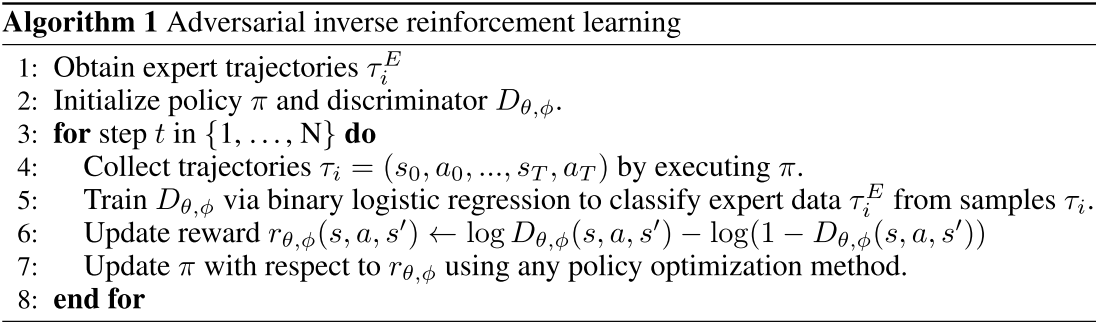# # Adversarial Inverse Reinforcement Learning

Learning Robust Rewards With Adversarial Inverse Reinforcement Learning

Justin Fu, Katie Luo, Sergey Levine

## # Abstract

Inverse reinforcement learning holds the promise of automatic reward acquisition, but has proven exceptionally difficult to apply to large, high-dimensional problems with unknown dynamics.

Dynamics是什么?

Known Dynamics指的就是知道这个状态转移概率,那么对应model-based; Unknown Dynamics指的是不知道这个转移概率, 那么对应model-free。

## # Introduction

Part of the challenge is that IRL is an ill-defined problem, since there are many optimal policies that can explain a set of demonstrations, and many rewards that can explain an optimal policy.

1. 许多最优策略可以用来解释专家的轨迹;
2. 许多的Reward Function可以用来解释最优策略;

## # Background

$p_{\theta}(\mathbf{x})=\frac{1}{Z} \exp \left(-E_{\theta}(\mathbf{x})\right)$

$D^{*}(\tau)=\frac{p(\tau)}{p(\tau)+q(\tau)}$

$D_{\theta}(\tau)=\frac{\tilde{p}_{\theta}(\tau)}{\tilde{p}_{\theta}(\tau)+q(\tau)}$

$D_{\theta}(\tau)=\frac{\frac{1}{Z} \exp \left(-c_{\theta}(\tau)\right)}{\frac{1}{Z} \exp \left(-c_{\theta}(\tau)\right)+q(\tau)}$

$D_{\theta}(\tau)=\frac{\exp \left\{f_{\theta}(\tau)\right\}}{\exp \left\{f_{\theta}(\tau)\right\}+\pi(\tau)}$

## # AIRL

$D_{\theta}(s, a)=\frac{\exp \left\{f_{\theta}(s, a)\right\}}{\exp \left\{f_{\theta}(s, a)\right\}+\pi(a | s)}$

1. 文章里面说的是他们学到的Reward Function之和State有关，那为什么表达式里面却是$r_{\theta,\phi} (s,a,s')$

2. 从后面的实验结果来看, 貌似这套算法的到的Reward Function是否只是和Statte相关还是可以任意切换的, 是怎么做到的?

Policy invariance under reward transformations: Theory and application to reward shaping. In International Conference on Machine Learning (ICML), 1999文章中提到在下面的Reward的变化中:

$\hat{r}\left(s, a, s^{\prime}\right)=r\left(s, a, s^{\prime}\right)+\gamma \Phi\left(s^{\prime}\right)-\Phi(s)$

## # 使用AIRL学习一个Disentangled Reward

$D_{\theta, \phi}\left(s, a, s^{\prime}\right)=\frac{\exp \left\{f_{\theta, \phi}\left(s, a, s^{\prime}\right)\right\}}{\exp \left\{f_{\theta, \phi}\left(s, a, s^{\prime}\right)\right\}+\pi(a | s)}$

$f_{\theta, \phi}\left(s, a, s^{\prime}\right)=g_{\theta}(s, a)+\gamma h_{\phi}\left(s^{\prime}\right)-h_{\phi}(s)$

$f_{\theta, \phi}\left(s, a,s^{\prime}\right)=g_{\theta}(s)+\gamma h_{\phi}\left(s^{\prime}\right)-h_{\phi}(s)$

$g^{*}(s)=r^{*}(s)+\mathrm{const}$

$h^{*}(s)=V^{*}(s)+\mathrm{const}$

$f^{*}\left(s, a, s^{\prime}\right)=r^{*}(s)+\gamma V^{*}\left(s^{\prime}\right)-V^{*}(s)=A^{*}(s, a)$$r_{\theta, \phi}\left(s, a, s^{\prime}\right) \leftarrow \log D_{\theta, \phi}\left(s, a, s^{\prime}\right)-\log \left(1-D_{\theta, \phi}\left(s, a, s^{\prime}\right)\right)$